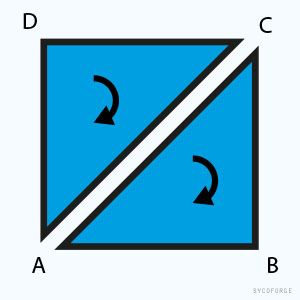# Creating mesh tiles from the terrain

I’d like to create multiple cells on a given terrain, and I want to use them as transparent meshes (to be activated when the player selects it).

The idea is to create these “tiles” with code (C#). How can I select these an area and create a meshes that adhere to the terrain?

The idea is to create hexagonal tiles, but for the sake of semplicity could you give me some hints to create square tiles?

(I’ll adjust the code to make it hexagonal).

This class lets you easily create a mesh from a specified terrain. Based on this class you can create what ever you want.

For larger terrains you have to split it to multiple meshes because a mesh can only hold 65000 vertices.

``````using System.Collections.Generic;
using UnityEngine;

public class TerrainMesher
{
/// <summary>
/// Converts a terrain to a mesh.
/// </summary>
/// <param name="terrain">The terrain to convert.</param>
/// <returns>The mesh</returns>
public static Mesh MeshFromTerrain(Terrain terrain)
{
// Create new mesh object
Mesh mesh = new Mesh();

TerrainData terrainData = terrain.terrainData;

// Create all lists needed
List<int> indices = new List<int>();
List<Vector3> vertices = new List<Vector3>();
List<Vector3> normals = new List<Vector3>();

Vector3 heightmapScale = terrainData.heightmapScale;

// Compute fractions in x and z direction
float dx = 1f / (terrainData.heightmapWidth - 1);
float dz = 1f / (terrainData.heightmapHeight - 1);

for (int ix = 0; ix < terrainData.heightmapWidth; ix++)
{
float x = ix * heightmapScale.x;
float ddx = ix * dx;

for (int iz = 0; iz < terrainData.heightmapHeight; iz++)
{
float z = iz * heightmapScale.z;
float ddz = iz * dz;

// Sample height and normal at dx, dz
Vector3 point = new Vector3(x, terrainData.GetInterpolatedHeight(ddx, ddz), z);
Vector3 normal = terrainData.GetInterpolatedNormal(ddx, ddz);

// Add vertex and normal to the lists
}
}

int w = terrainData.heightmapWidth;
int h = terrainData.heightmapHeight;

for (int xx = 0; xx < w-1; xx++)
{
for (int zz = 0; zz < h-1; zz++)
{
int a = zz + xx * w;
int b = a + w + 1;
int c = a + w;
int d = a + 1;

// Add indices in clockwise order (winding order)

}
}

mesh.vertices = vertices.ToArray();
mesh.normals = normals.ToArray();
mesh.triangles = indices.ToArray();

// Recalculate mesh bounds
mesh.RecalculateBounds();

return mesh;
}
}
``````

The triangles are constructed as shown on the image below.